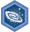## FANDOM

1,895 Pages

Algebra II: Rational expressions, equations, and functionsDescription
Achieve mastery in all skills in Algebra II: Rational expressions, equations, and functions
Details
TypeEnergy point(s) awarded 0
Status Available
Disclosed?
Repeating?

This page contains exercises which pertain to the subcategory Algebra II: Rational expressions, equations, and functions. Mastering them awards user with the Challenge Patch Algebra II: Rational expressions, equations, and functions as well.

All items (41)

A
•Add and subtract rational expressions by finding the least common multiple
•Add and subtract rational expressions with factored denominators
•Add and subtract rational expressions with relatively prime denominators
•Add and subtract rational expressions with the same denominator
•Adding and subtracting rational expressions 1
•Adding and subtracting rational expressions 2
•Adding and subtracting rational expressions 3
•Adding and subtracting rational expressions 4
•Adding and subtracting rational expressions 5
•Adding and subtracting rational expressions 6
•Adding and subtracting rational expressions 7
D
•Determine the end behavior of rational functions
•Distinguish between direct and inverse variation
•Dividing polynomials by binomials 1
•Dividing polynomials by binomials 2
•Dividing polynomials by binomials 3
E
•Extraneous solutions to rational equations
F
•Factor perfect squares
G
•Graphs of rational functions
I
•Identify and analyze discontinuities of rational functions
M
•Match graphs of rational functions to their formula
•Multiply and divide advanced rational expressions
•Multiply and divide rational expressions with monomial numerators and denominators
•Multiply and divide rational expressions with polynomial numerators and denominators
•Multiplying and dividing rational expressions 1
•Multiplying and dividing rational expressions 2
•Multiplying and dividing rational expressions 3
•Multiplying and dividing rational expressions 4
•Multiplying and dividing rational expressions 5
P
•Partial fraction expansion
S
•Simplify rational expressions by canceling binomial factors
•Simplify rational expressions by canceling monomial factors
•Simplify rational expressions using advanced factorization methods
•Simplifying rational expressions 2
•Simplifying rational expressions 3
•Simplifying rational expressions 4
•Solve equations with one rational expression (advanced)
•Solve equations with one rational expression (basic)
•Solve equations with two rational expressions
•Solving rational equations 1
•Solving rational equations 2